# R S Aggarwal Solutions for Class 10 Maths Exercise 18B Chapter 18 Mean, Median, Mode of Grouped Data, Cumulative Frequency Graph and Ogive

R S Aggarwal Solutions for Class 10 Maths exercise 18B is available here. All long and short types questions from the textbooks are answered in detail for your reference. It helps to accelerate your knowledge on the median for grouped data using formula. Students are advised to download Class 10 Maths Chapter 18 R S Aggarwal Solutions and score well in examinations.

## Download PDF of R S Aggarwal Solutions for Class 10 Maths Chapter 18 Mean, Median, Mode of Grouped Data, Cumulative Frequency Graph and Ogive Exercise 18B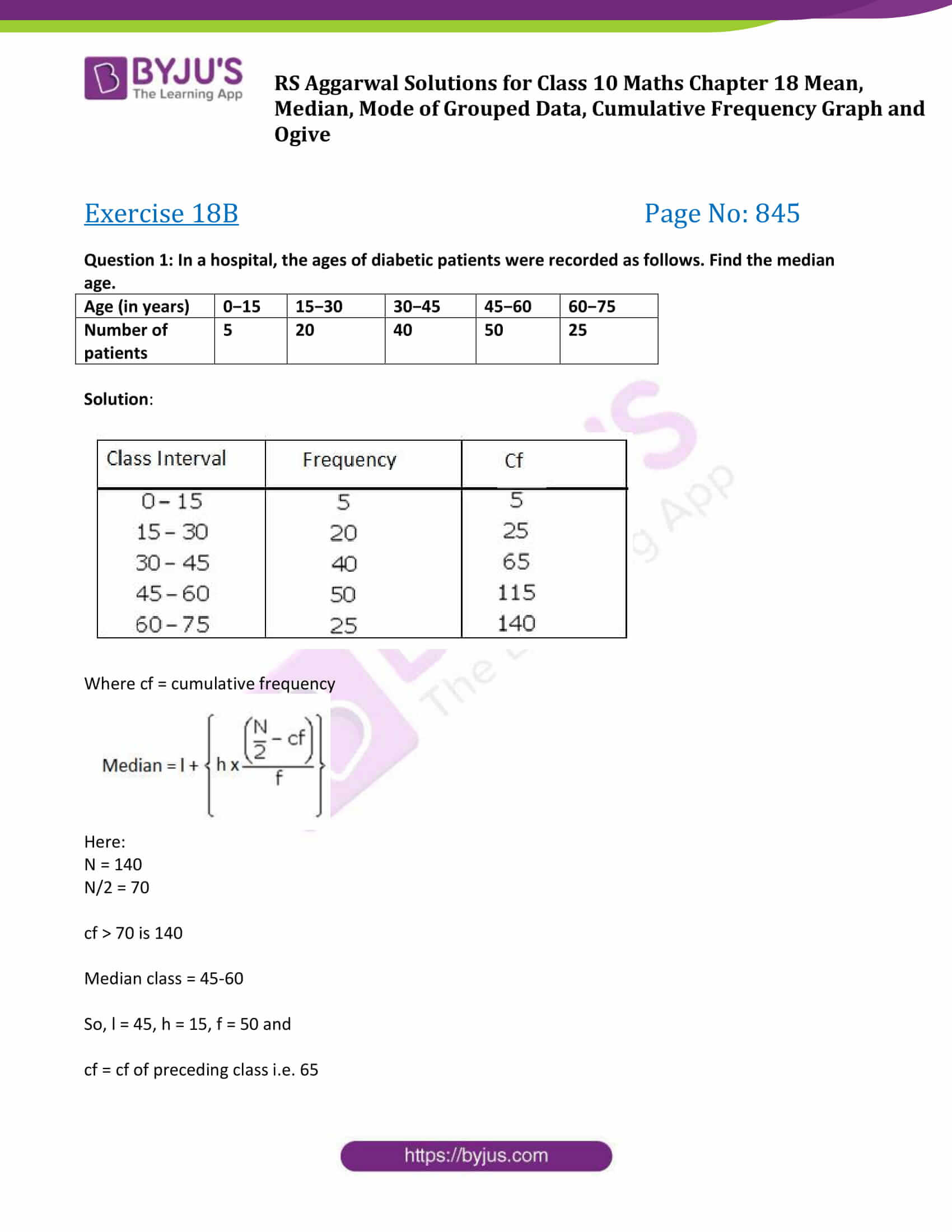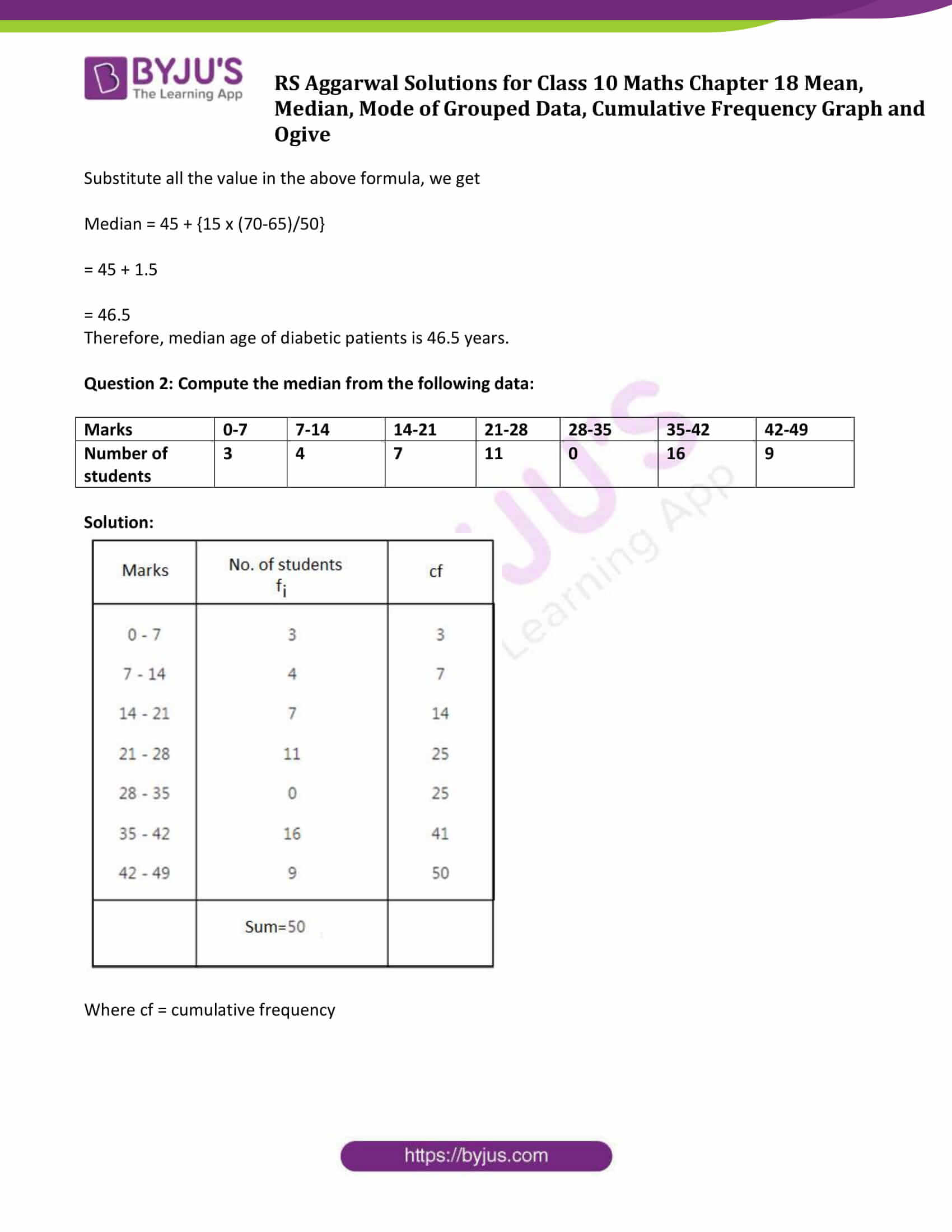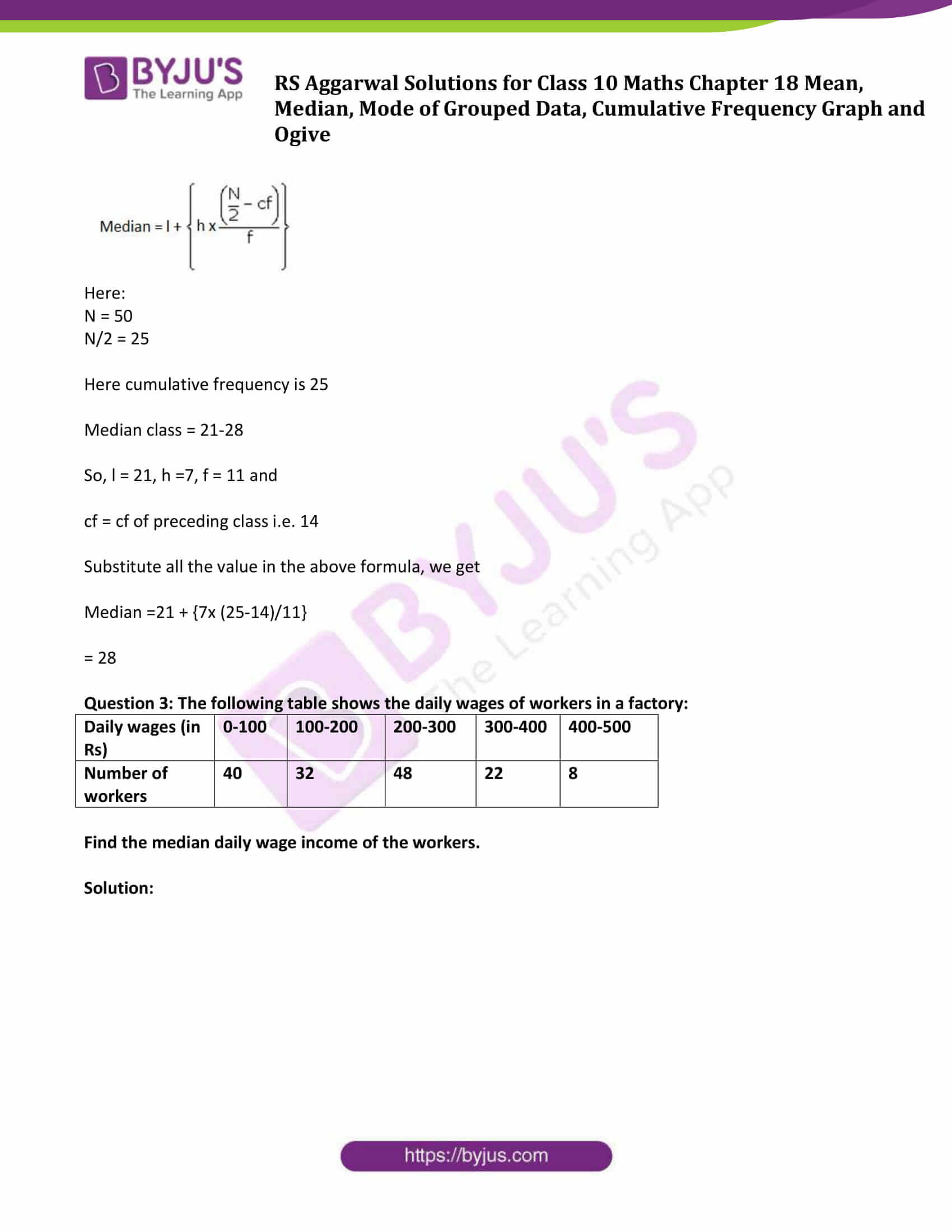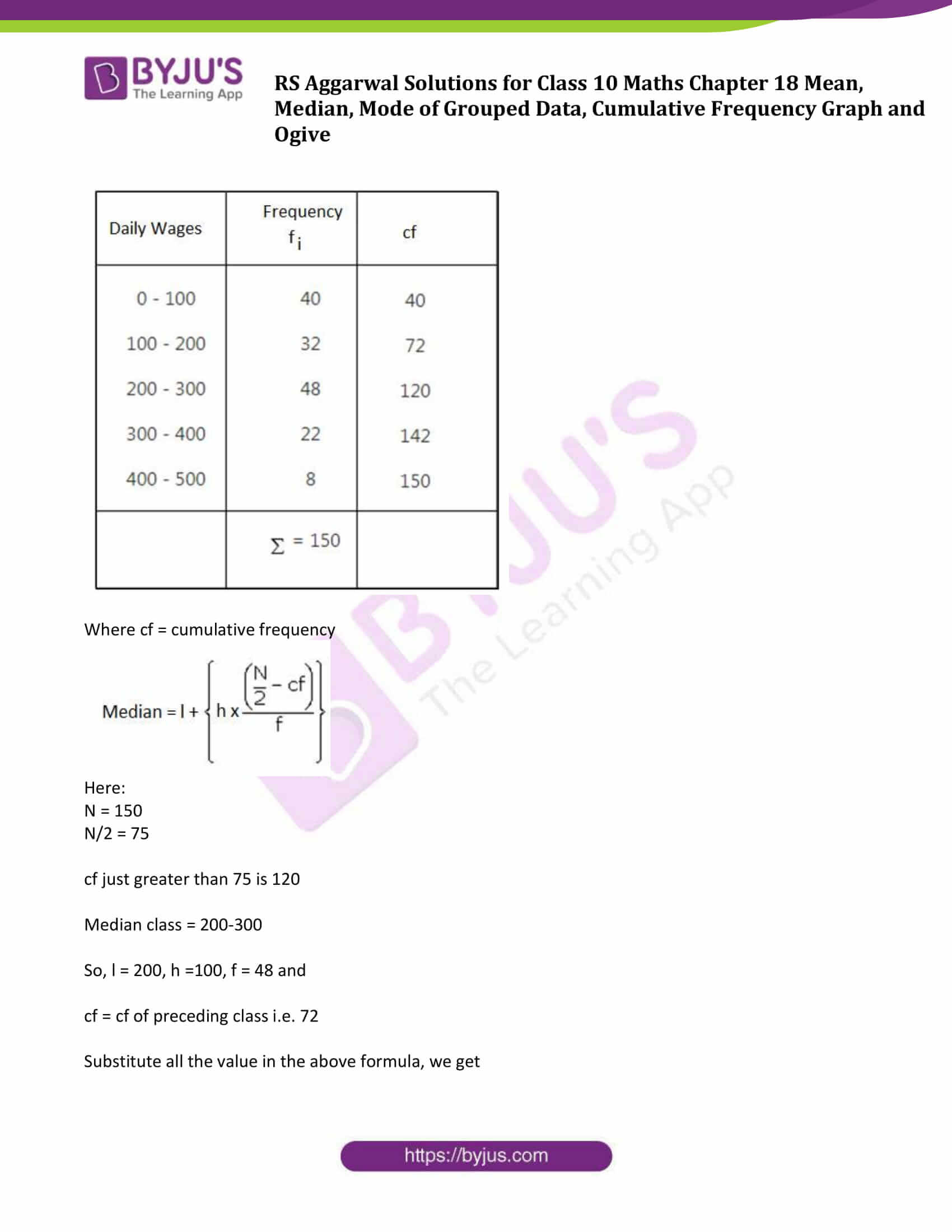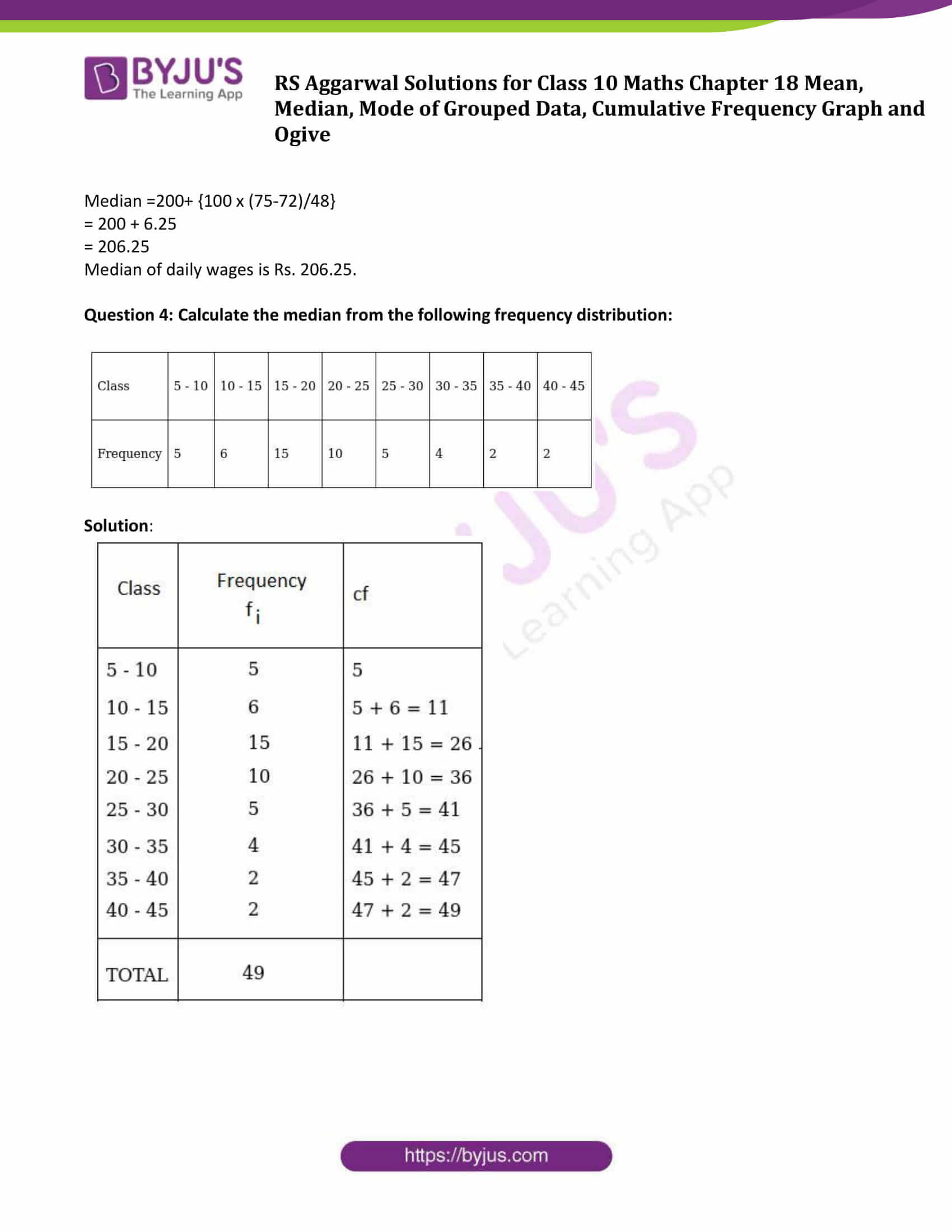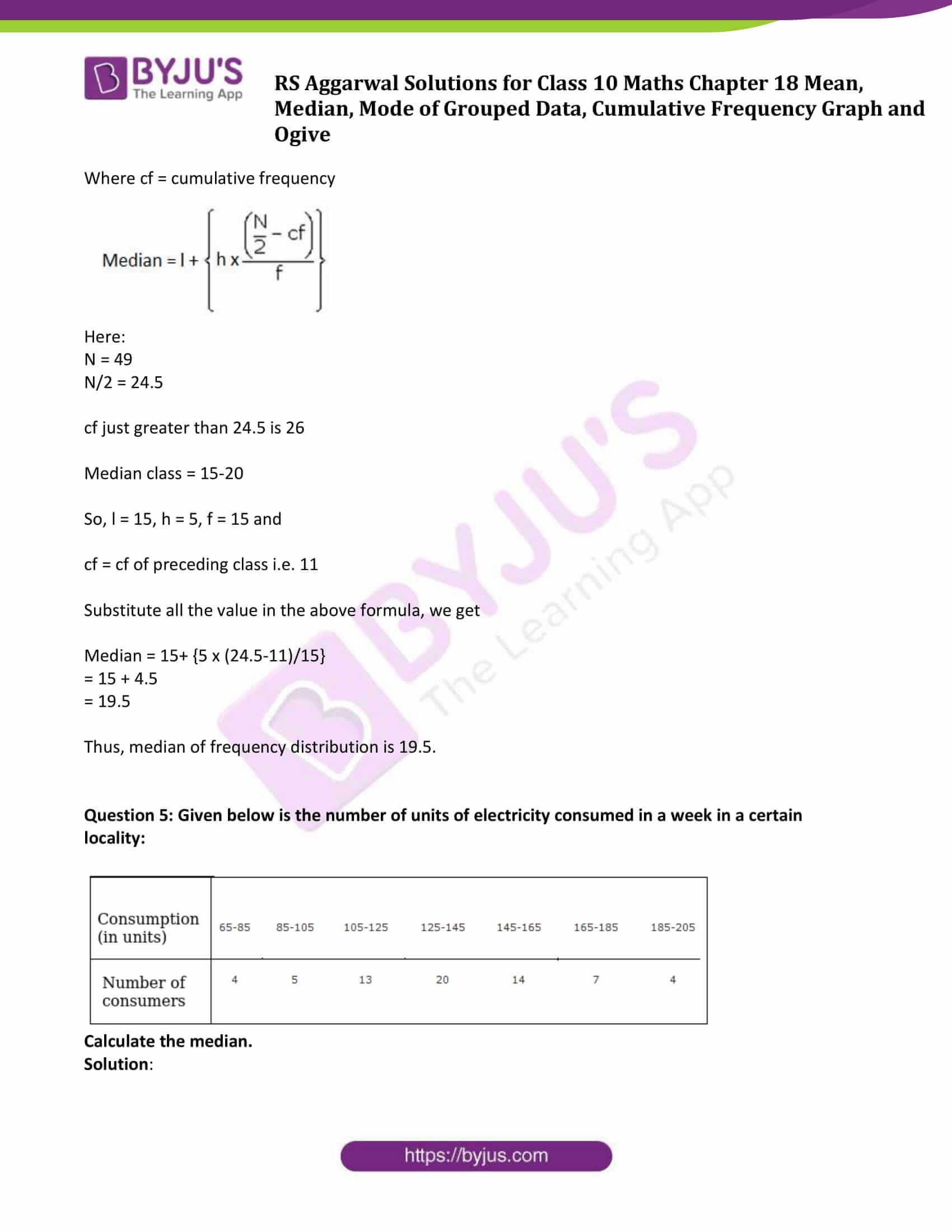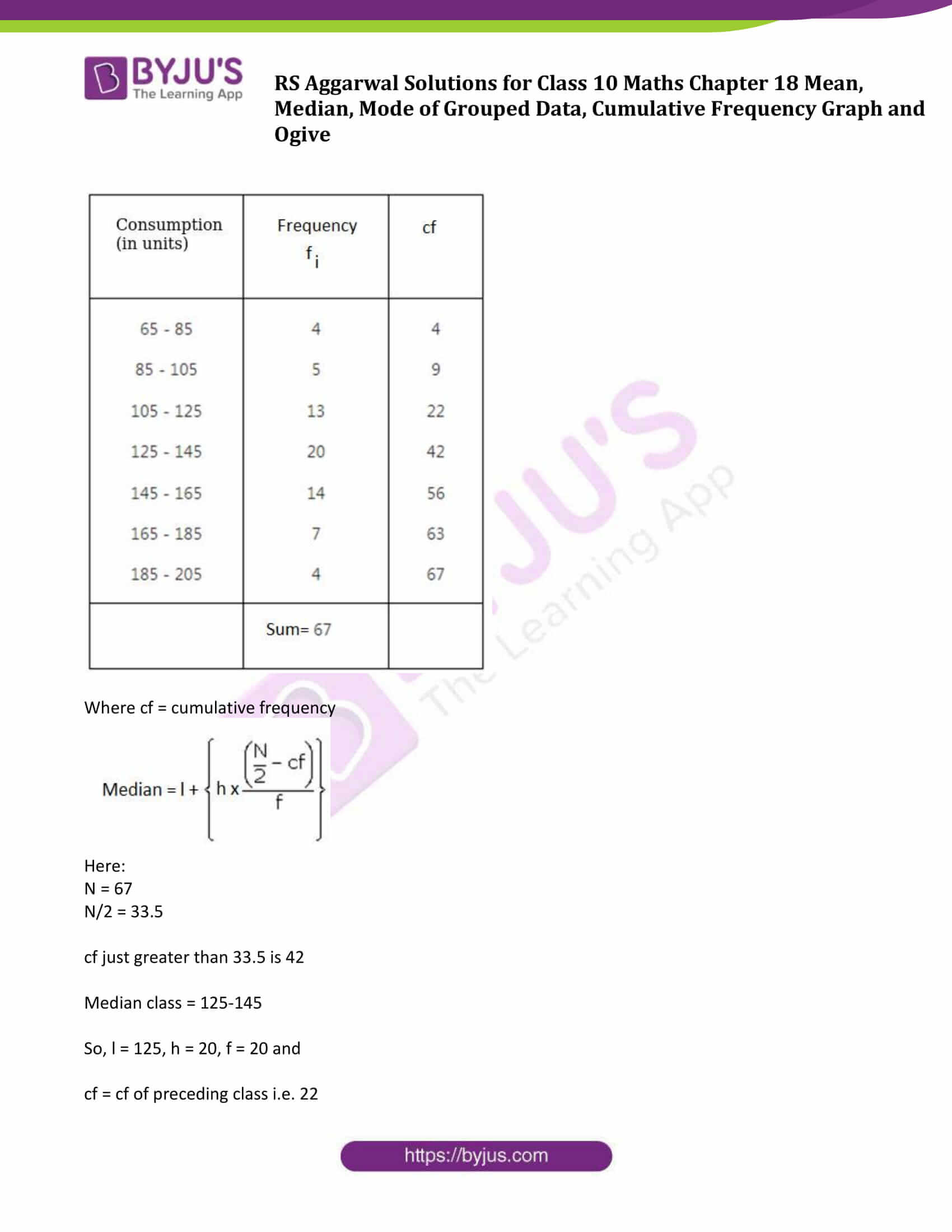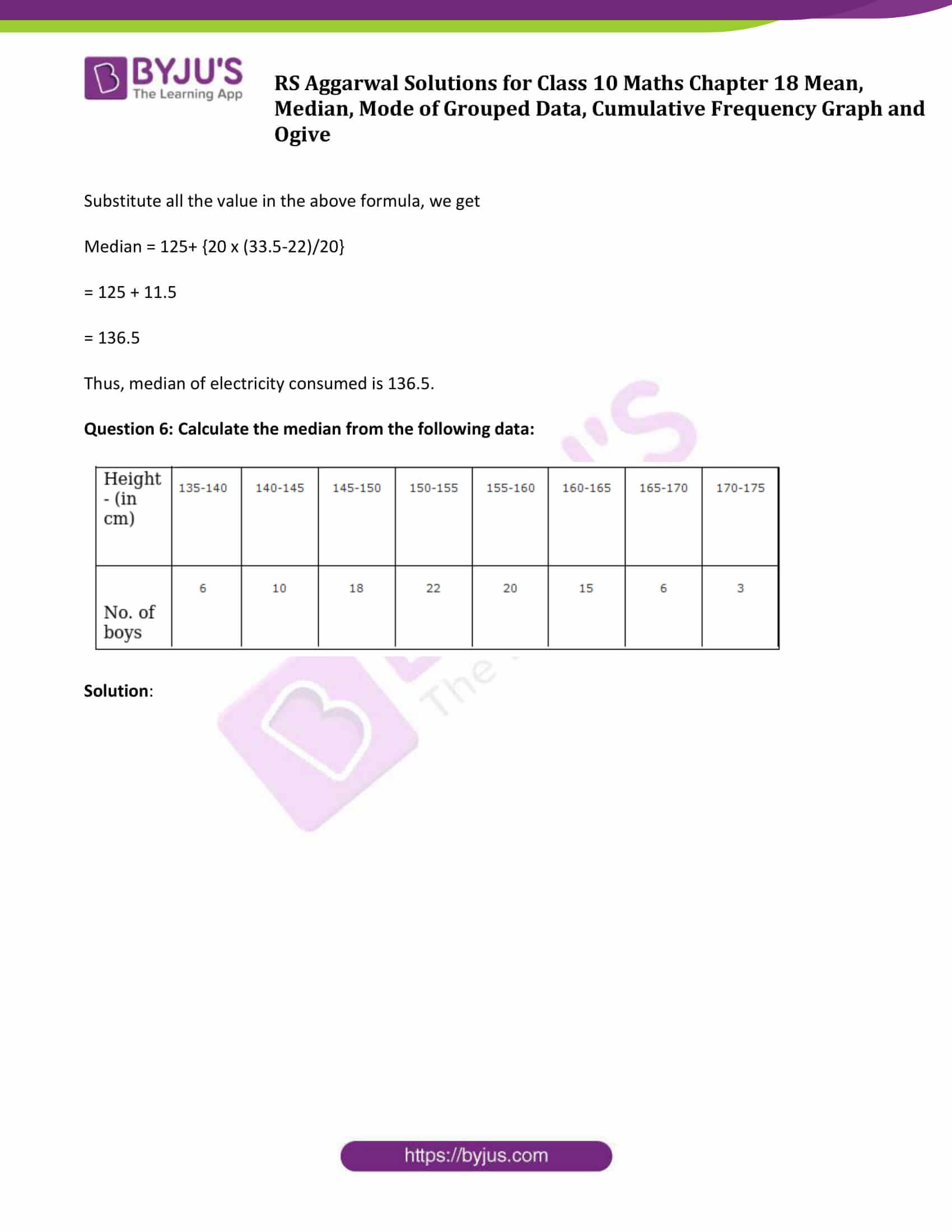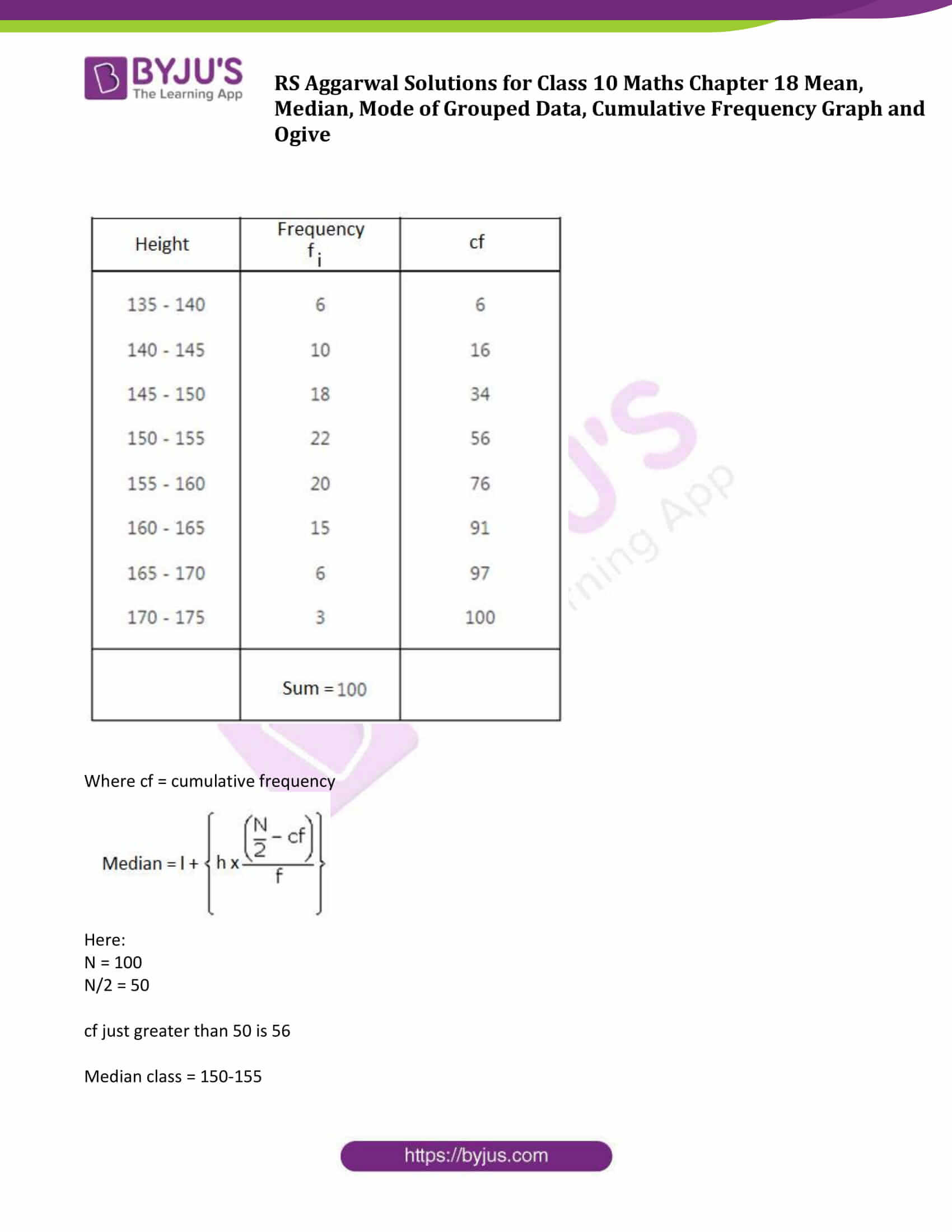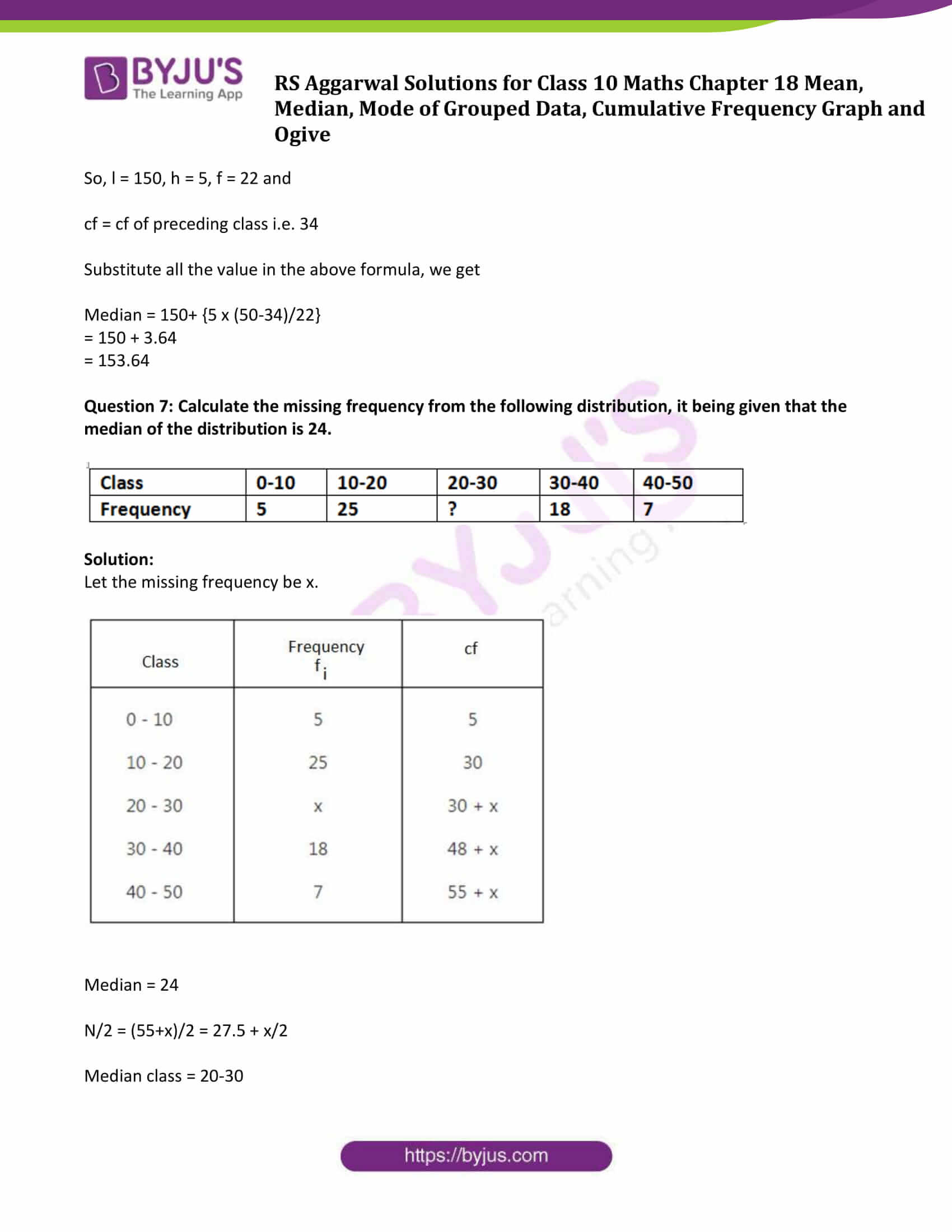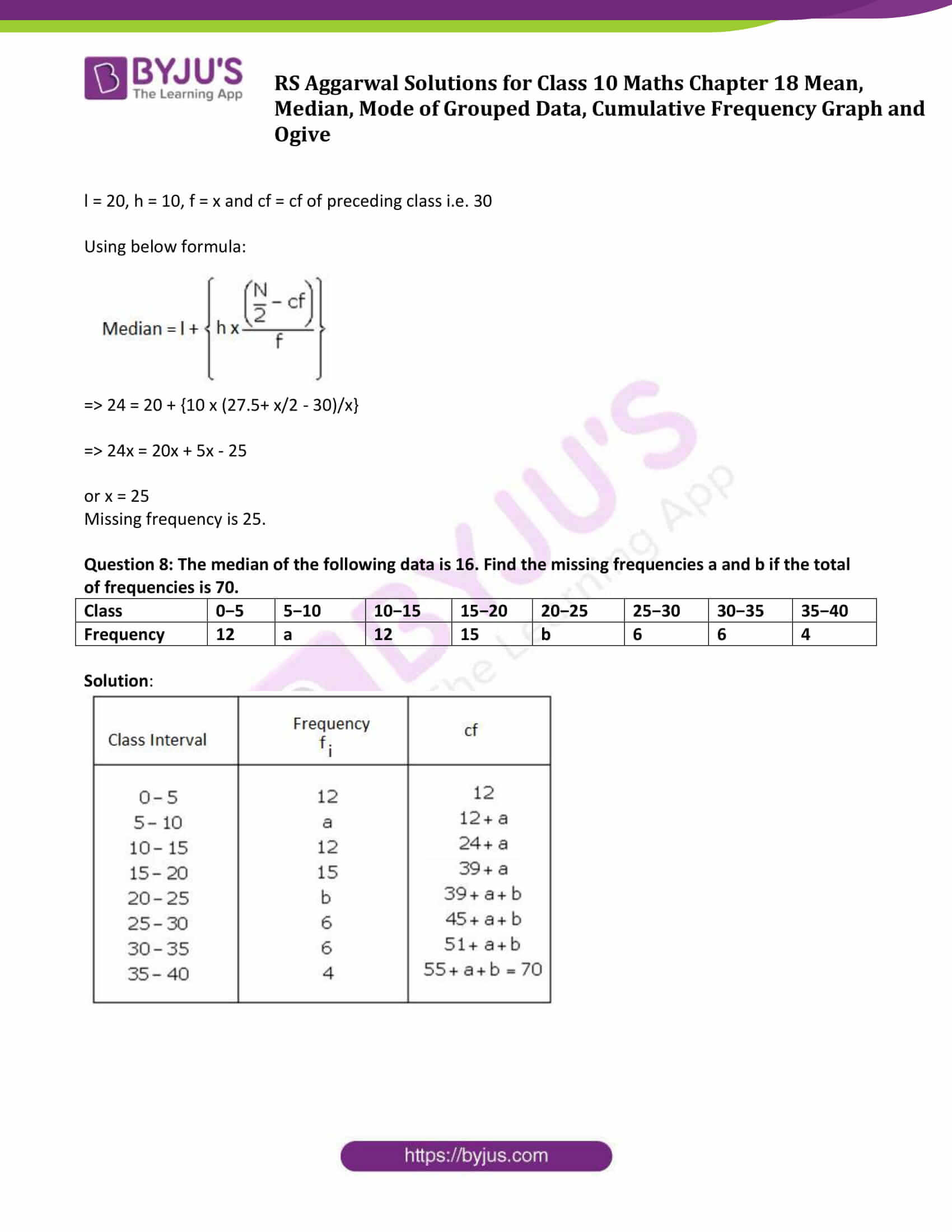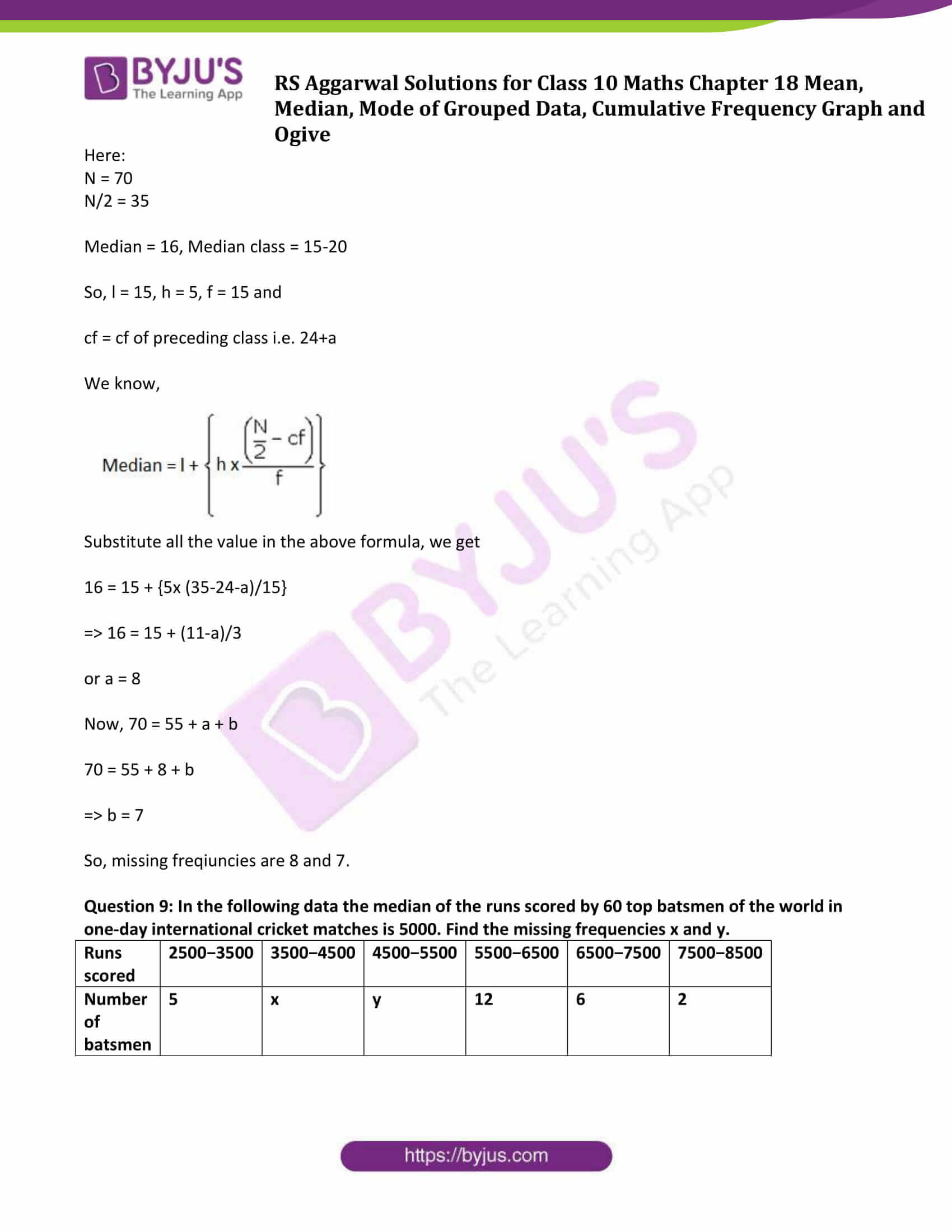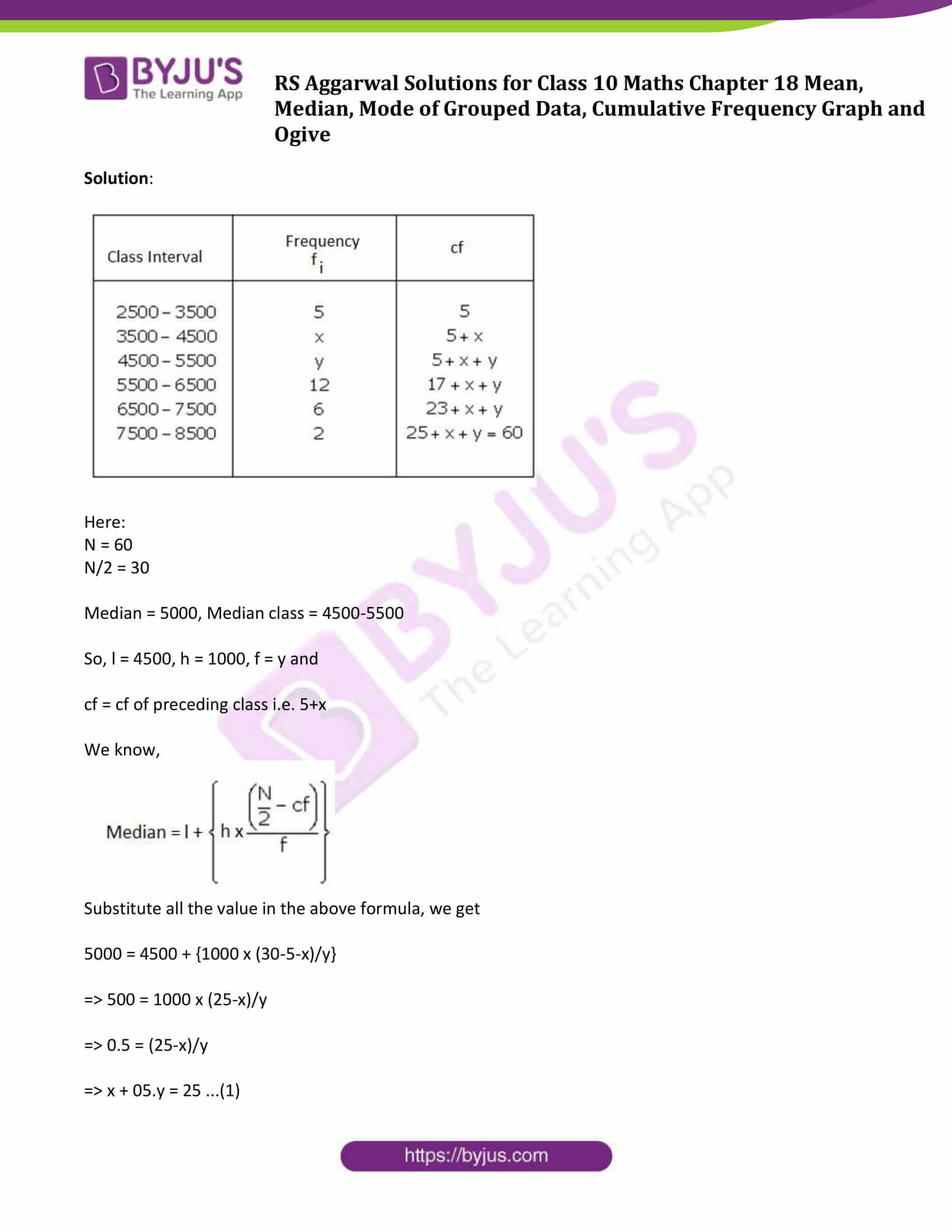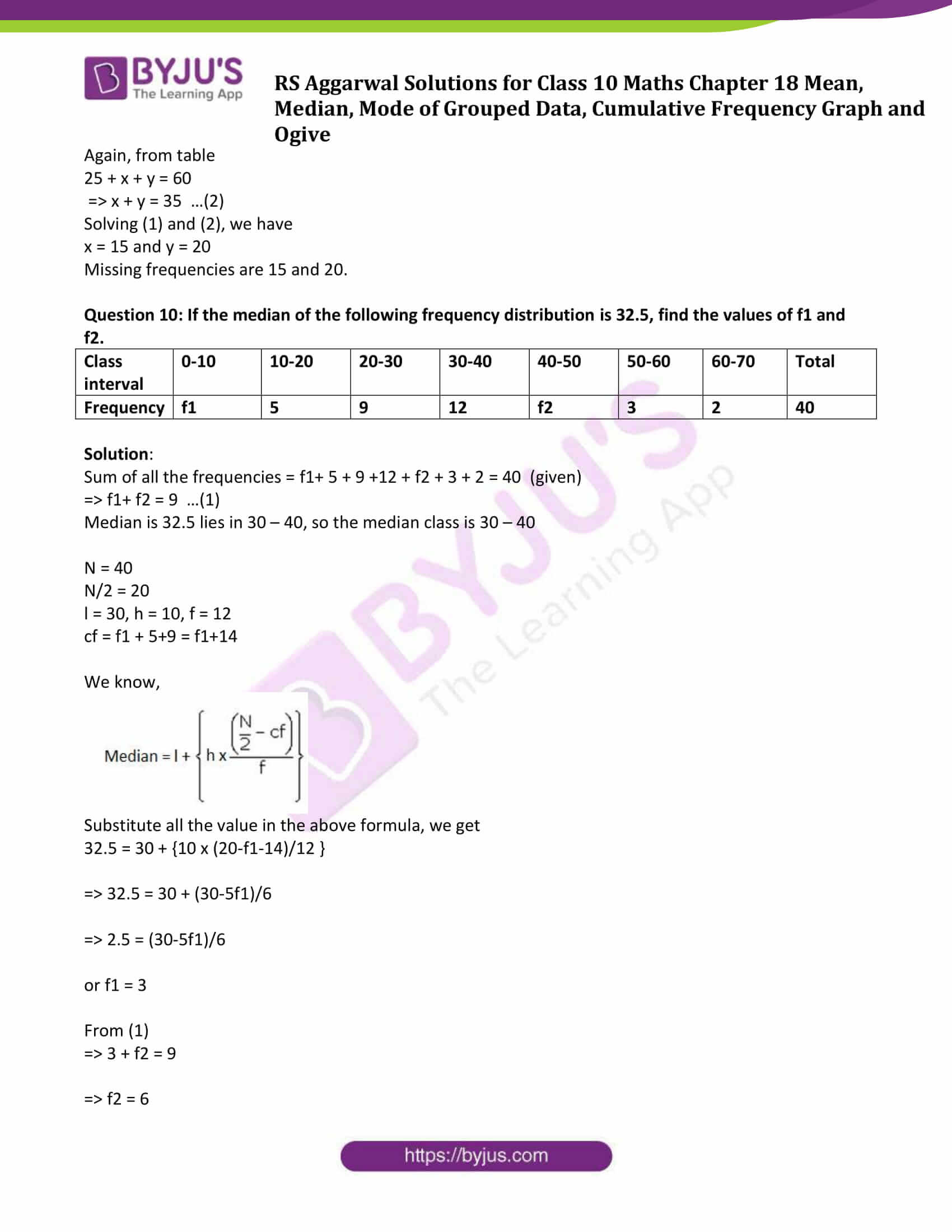## Access other exercise solutions of Class 10 Maths Chapter 18 Mean, Median, Mode of Grouped Data, Cumulative Frequency Graph and Ogive

Exercise 18 A Solutions

Exercise 18 C Solutions

Exercise 18 D Solutions

Exercise 18 E Solutions

## Exercise 18B

Question 1: In a hospital, the ages of diabetic patients were recorded as follows. Find the median age.

 Age (in years) 0−15 15−30 30−45 45−60 60−75 Number of patients 5 20 40 50 25

Solution: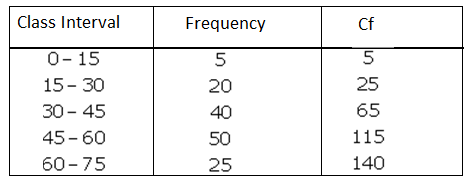Where cf = cumulative frequency

$Median = I +\left \{ h\times\frac{\left ( \frac{N}{2} – cf\right )}{f} \right \}$

Here:

N = 140

N/2 = 70

cf > 70 is 140

Median class = 45-60

So, l = 45, h = 15, f = 50 and

cf = cf of preceding class i.e. 65

Substitute all the value in the above formula, we get

Median = 45 + {15 x (70-65)/50}

= 45 + 1.5

= 46.5

Therefore, median age of diabetic patients is 46.5 years.

Question 2: Compute the median from the following data:

 Marks 0-7 7-14 14-21 21-28 28-35 35-42 42-49 Number of students 3 4 7 11 0 16 9

Solution: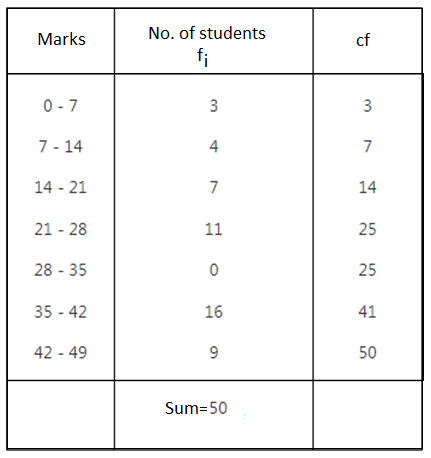Where cf = cumulative frequency

$Median = I +\left \{ h\times\frac{\left ( \frac{N}{2} – cf\right )}{f} \right \}$

Here:

N = 50

N/2 = 25

Here cumulative frequency is 25

Median class = 21-28

So, l = 21, h =7, f = 11 and

cf = cf of preceding class i.e. 14

Substitute all the value in the above formula, we get

Median =21 + {7x (25-14)/11}

= 28

Question 3: The following table shows the daily wages of workers in a factory:

 Daily wages (in Rs) 0-100 100-200 200-300 300-400 400-500 Number of workers 40 32 48 22 8

Find the median daily wage income of the workers.

Solution: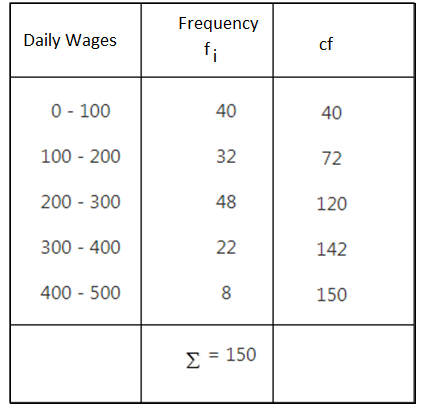Where cf = cumulative frequency

$Median = I +\left \{ h\times\frac{\left ( \frac{N}{2} – cf\right )}{f} \right \}$

Here:

N = 150

N/2 = 75

cf just greater than 75 is 120

Median class = 200-300

So, l = 200, h =100, f = 48 and

cf = cf of preceding class i.e. 72

Substitute all the value in the above formula, we get

Median =200+ {100 x (75-72)/48}

= 200 + 6.25

= 206.25

Median of daily wages is Rs. 206.25.

Question 4: Calculate the median from the following frequency distribution: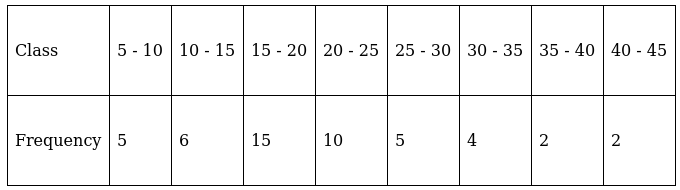Solution: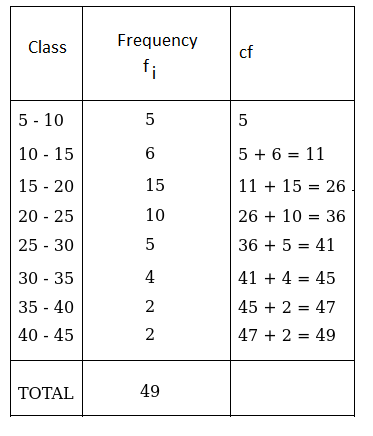Where cf = cumulative frequency

$Median = I +\left \{ h\times\frac{\left ( \frac{N}{2} – cf\right )}{f} \right \}$

Here:

N = 49

N/2 = 24.5

cf just greater than 24.5 is 26

Median class = 15-20

So, l = 15, h = 5, f = 15 and

cf = cf of preceding class i.e. 11

Substitute all the value in the above formula, we get

Median = 15+ {5 x (24.5-11)/15}

= 15 + 4.5

= 19.5

Thus, median of frequency distribution is 19.5.

Question 5: Given below is the number of units of electricity consumed in a week in a certain locality: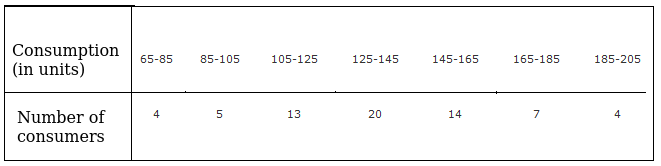Calculate the median.

Solution: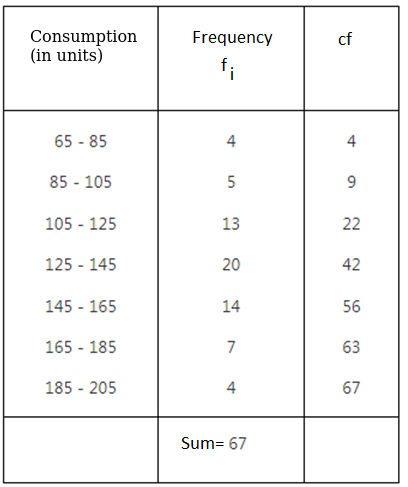Where cf = cumulative frequency

$Median = I +\left \{ h\times\frac{\left ( \frac{N}{2} – cf\right )}{f} \right \}$

Here:

N = 67

N/2 = 33.5

cf just greater than 33.5 is 42

Median class = 125-145

So, l = 125, h = 20, f = 20 and

cf = cf of preceding class i.e. 22

Substitute all the value in the above formula, we get

Median = 125+ {20 x (33.5-22)/20}

= 125 + 11.5

= 136.5

Thus, median of electricity consumed is 136.5.

Question 6: Calculate the median from the following data: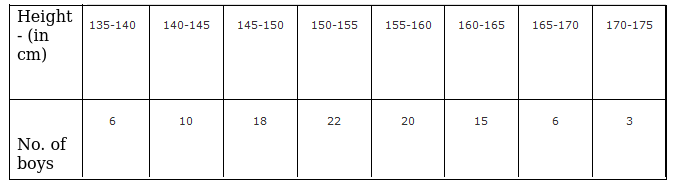Solution: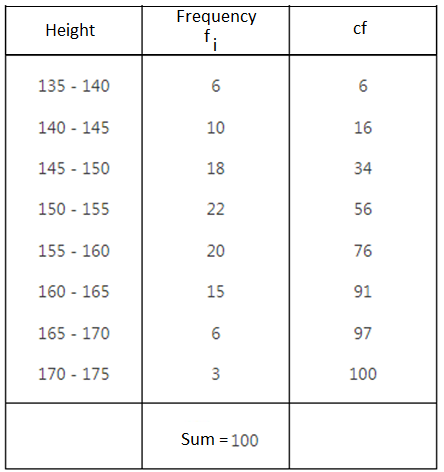Where cf = cumulative frequency

$Median = I +\left \{ h\times\frac{\left ( \frac{N}{2} – cf\right )}{f} \right \}$

Here:

N = 100

N/2 = 50

cf just greater than 50 is 56

Median class = 150-155

So, l = 150, h = 5, f = 22 and

cf = cf of preceding class i.e. 34

Substitute all the value in the above formula, we get

Median = 150+ {5 x (50-34)/22}

= 150 + 3.64

= 153.64

Question 7: Calculate the missing frequency from the following distribution, it being given that the median of the distribution is 24.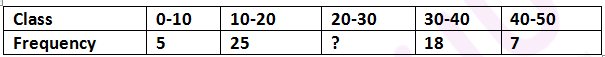Solution:

Let the missing frequency be x.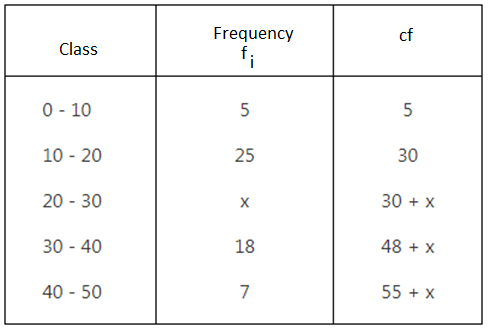Median = 24

N/2 = (55+x)/2 = 27.5 + x/2

Median class = 20-30

l = 20, h = 10, f = x and cf = cf of preceding class i.e. 30

Using below formula:

$Median = I +\left \{ h\times\frac{\left ( \frac{N}{2} – cf\right )}{f} \right \}$

⇨ 24 = 20 + {10 x (27.5+ x/2 – 30)/x}

⇨ 24x = 20x + 5x – 25

or x = 25

Missing frequency is 25.

Question 8: The median of the following data is 16. Find the missing frequencies a and b if the total of frequencies is 70.

 Class 0−5 5−10 10−15 15−20 20−25 25−30 30−35 35−40 Frequency 12 a 12 15 b 6 6 4

Solution: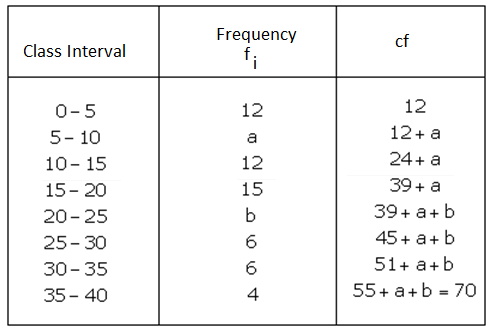Here:

N = 70

N/2 = 35

Median = 16, Median class = 15-20

So, l = 15, h = 5, f = 15 and

cf = cf of preceding class i.e. 24+a

We know,

$Median = I +\left \{ h\times\frac{\left ( \frac{N}{2} – cf\right )}{f} \right \}$

Substitute all the value in the above formula, we get

16 = 15 + {5x (35-24-a)/15}

⇨ 16 = 15 + (11-a)/3

or a = 8

Now, 70 = 55 + a + b

70 = 55 + 8 + b

⇨ b = 7

So, missing freqiuncies are 8 and 7.

Question 9: In the following data the median of the runs scored by 60 top batsmen of the world in one-day international cricket matches is 5000. Find the missing frequencies x and y.

 Runs scored 2500−3500 3500−4500 4500−5500 5500−6500 6500−7500 7500−8500 Number of batsmen 5 x y 12 6 2

Solution: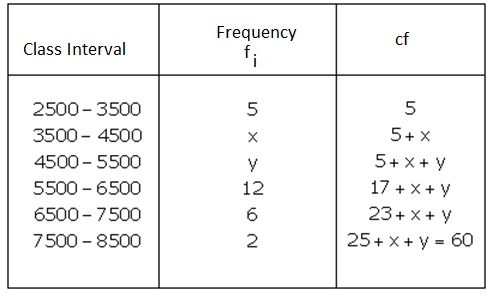Here:

N = 60

N/2 = 30

Median = 5000, Median class = 4500-5500

So, l = 4500, h = 1000, f = y and

cf = cf of preceding class i.e. 5+x

We know,

$Median = I +\left \{ h\times\frac{\left ( \frac{N}{2} – cf\right )}{f} \right \}$

Substitute all the value in the above formula, we get

5000 = 4500 + {1000 x (30-5-x)/y}

⇨ 500 = 1000 x (25-x)/y

⇨ 0.5 = (25-x)/y

⇨ x + 05.y = 25 …(1)

Again, from table

25 + x + y = 60

⇨ x + y = 35 …(2)

Solving (1) and (2), we have

x = 15 and y = 20

Missing frequencies are 15 and 20.

Question 10: If the median of the following frequency distribution is 32.5, find the values of f1 and f2.

 Class interval 0-10 10-20 20-30 30-40 40-50 50-60 60-70 Total Frequency f1 5 9 12 f2 3 2 40

Solution:

Sum of all the frequencies = f1+ 5 + 9 +12 + f2 + 3 + 2 = 40 (given)

⇨ f1+ f2 = 9 …(1)

Median is 32.5 lies in 30 – 40, so the median class is 30 – 40

N = 40

N/2 = 20

l = 30, h = 10, f = 12

cf = f1 + 5+9 = f1+14

We know,

$Median = I +\left \{ h\times\frac{\left ( \frac{N}{2} – cf\right )}{f} \right \}$

Substitute all the value in the above formula, we get

32.5 = 30 + {10 x (20-f1-14)/12 }

⇨ 32.5 = 30 + (30-5f1)/6

⇨ 2.5 = (30-5f1)/6

or f1 = 3

From (1)

⇨ 3 + f2 = 9

⇨ f2 = 6

## R S Aggarwal Solutions for Class 10 Maths Chapter 18 Mean, Median, Mode of Grouped Data, Cumulative Frequency Graph and Ogive Exercise 18B Topics:

Class 10 Maths Chapter 18 Mean, Median, Mode of Grouped Data, Cumulative Frequency Graph and Ogive Exercise 18B is based on the median for grouped data

Formula to find median:

$Median = I +\left \{ h\times\frac{\left ( \frac{N}{2} – cf\right )}{f} \right \}$

where:

l = lower class boundary

N = total number of values

cf = cumulative frequency of the groups before the median group

f = frequency of the median group

h = the group width